Courses

# NCERT Exemplar - Structure of Atom JEE Notes | EduRev

## JEE : NCERT Exemplar - Structure of Atom JEE Notes | EduRev

The document NCERT Exemplar - Structure of Atom JEE Notes | EduRev is a part of the JEE Course JEE Revision Notes.
All you need of JEE at this link: JEE

MULTIPLE CHOICE QUESTIONS - I

Q.1. Which of the following conclusions could not be derived from Rutherford’s α -particle scattering experiment?
(1) Most of the space in the atom is empty.
(2) The radius of the atom is about 10–10 m while that of nucleus is 10–15 m.
(3) Electrons move in a circular path of fixed energy called orbits.
(4) Electrons and the nucleus are held together by electrostatic forces of attraction
Ans.
(3)
Solution.

The concept of circular paths of fixed energy was proposed by Bohr and not derived from Rutherford’s scattering experiment.

Q.2. Which of the following options does not represent ground state electronic configuration of an atom?
(1) 1s2 2s2 2p6 3s2 3p6 3d8 4s2
(2) 1s2 2s2 2p6 3s2 3p6 3d9 4s2
(3) 1s2 2s2 2p6 3s2 3p6 3d10 4s1
(4) 1s2 2s2 2p6 3s2 3p6 3d5 4s1
Ans.
(2)
Solution.
Correct electronic configuration of the two subshells (3d and 4s) should be 3d104s1.
In certain elements such as Cu or Cr, where the two subshells (4s and 3d) differ slightly in their energies, an electron shifts from a subshell of lower energy (4s) to a subshell of higher energy (3d), provided such a shift results in all orbitals of the subshell of higher energy getting either completely filled or half filled.

Q.3.  The probability density plots of 1s and 2s orbitals are given in Figure: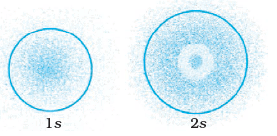The density of dots in a region represents the probability density of finding electrons in the region.
On the basis of above diagram which of the following statements is incorrect?
(1) 1s and 2s orbitals are spherical in shape.
(2) The probability of finding the electron is maximum near the nucleus.
(3) The probability of finding the electron at a given distance is equal in all directions.
(4) The probability density of electrons for 2s orbital decreases uniformly as distance from the nucleus increases
Ans.
(4)
Solution.
The probability density of electrons for 2s orbital first increases then decreases and after that it begins to increase again.

Q.4. Which of the following statement is not correct about the characteristics of cathode rays?
(1) They start from the cathode and move towards the anode.
(2) They travel in straight line in the absence of an external electrical or magnetic field.
(3) Characteristics of cathode rays do not depend upon the material of electrodes in cathode ray tube.
(4) Characteristics of cathode rays depend upon the nature of gas present in the cathode ray tube.
Ans.
(4)
Solution.
Cathode rays consist of negatively charged material particles called electron. They were discovered by William Crookes. The characteristics of cathode rays do not depend upon the material of electrodes and the nature of the gas present in the cathode ray tube.

Q.5. Which of the following statements about the electron is incorrect?
(1) It is a negatively charged particle.
(2) The mass of electron is equal to the mass of neutron.
(3) It is a basic constituent of all atoms.
(4) It is a constituent of cathode rays
Ans.
(2)
Solution.

The mass of electron is very small as compared to the mass of the neutron. Mass of electron = 9.1 x 10-31 kg Mass of neutron = 1.674 x 10-27 kg

Q.6. Which of the following properties of atom could be explained correctly by Thomson Model of atom?
(1) Overall neutrality of atom
(2) Spectra of hydrogen atom
(3) Position of electrons, protons and neutrons in atom
(4) Stability of atom
Ans. (1)

Solution.

According to Thomson model of atom, the mass of the atom is assumed to be uniformly distributed over the atom. This model was able to explain the overall neutrality of the atom.

Q.7. Two atoms are said to be isobars if.
(1) They have same atomic number but different mass number
(2) They have same number of electrons but different number of neutrons
(3) They have same number of neutrons but different number of electrons
(4) Sum of the number of protons and neutrons is same but the number of protons is different
Ans.
(4)
Solution.
Isobars have different atomic number, i.e., number of protons but same mass number, i.e., sum of number of protons and neutrons.

Q.8. The number of radial nodes for 3p orbital is __________.
(1) 3
(2) 4
(3) 2
(4) 1
Ans.
(4)
Solution.
Number of radial nodes = n-1 – 1
For 3p orbital, n = 3 – 1 – 1 = 1
Number of radial nodes = 3 – 1 – 1 = 1

Q.9. Number of angular nodes for 4d orbital is __________.
(1) 4
(2) 3
(3) 2
(4) 1
Ans.
(3)
Solution.
Number of angular nodes in 4d orbital = l = 2

Q.10. Which of the following is responsible to rule out the existence of definite paths or trajectories of electrons?
(1) Pauli’s exclusion principle.
(2) Heisenberg’s uncertainty principle.
(3) Hund’s rule of maximum multiplicity.
(4) Aufbau principle.
Ans.
(2)
Solution.
According to Heisenberg’s uncertainty principle, the position and velocity of an electron cannot be determined simultaneously with accuracy which rules out the existence of fixed paths.

Q.11. Total number of orbitals associated with third shell will be __________.
(1) 2
(2) 4
(3) 9
(4) 3
Ans.
(3)
Solution.
No of orbitals in 3rd shell (n = 3) = n2 = 32 = 9.

Q.12. Orbital angular momentum depends on __________.
(1) l
(2) n and l
(3) n and m
(4) m and s
Ans.
(1)
Solution.
'I' also known as orbital angular momentum or subsidiary quantum number. It defines the three dimensional shape of the orbital. For a given value of n, l can have n values ranging from 0 to n-1, that is, for a given value of n, the possible values of l are: l=-0,1,2 ........ (n-1).

Q.13. Chlorine exists in two isotopic forms, Cl-37 and Cl-35 but its atomic mass is 35.5. This indicates the ratio of Cl-37 and Cl-35 is approximately
(1) 1:2
(2) 1:1
(3) 1:3
(4) 3:1
Ans.
(3)
Solution.

Ordinary chlorine is a mixture of two isotopes with atomic masses 37U and
35U and they are present in ratio 1:3
Hence, average atomic mass  =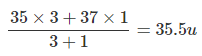Q.14. The pair of ions having same electronic configuration is __________.
(1) Cr3+, Fe3+
(2) Fe3+, Mn2+
(3) Fe3+, Co3+
(4) Sc3+, Cr3+
Ans.
(2)
Solution.

Fe(Z = 26) and Mn(Z = 25)
Fe3+ and Mn2+ will have same no. of electrons and hence, the same electronic configuration.

Q.15. For the electrons of oxygen atom, which of the following statements is correct?
(1) Zeff for an electron in a 2s orbital is the same as Zeff for an electron in a 2p orbital
(2) An electron in the 2s orbital has the same energy as an electron in the 2p orbital
(3) Zeff for an electron in 1s orbital is the same as Zeff for an electron in a 2s orbital
(4) The two electrons present in the 2s orbital have spin quantum numbers ms but of opposite sign
Ans.
(4)
Solution.

Pauli Exclusion Principle: Only two electrons may exist in the same orbital and
these electrons must have opposite spin.

Q.16. If travelling at same speeds, which of the following matter waves have the shortest wavelength?
(1) Electron
(2) Alpha particle (He2+)
(3) Neutron
(4) Proton
Ans.
(2)
Solution.
λ = h/mv = h/p
Higher the mass, shortest is the wavelength. Since alpha particles have the highest mass, they have shortest wavelength.

MULTIPLE CHOICE QUESTIONS - I

In the following questions two or more options may be correct.
Q. 17. Identify the pairs which are not of isotopes?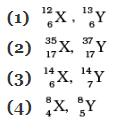Ans. (3,4)
Solution.
Isotopes have the same atomic number but different mass number.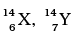and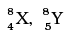are not isotopes.

Q.18. Out of the following pairs of electrons,  identify the pairs of electrons present in degenerate orbitals:
(1)
(a) n = 3, l = 2, ml = –2,   ms = -1/2
(b) n = 3, l = 2, ml = –1,   ms = -1/2
(2)
(a) n = 3, l = 1, ml = 1, m = +1/2
(b) n = 3, l = 2, ml = 1, ms = +1/2
(3)
(a) n = 4, l = 1, ml = 1, ms = +1/2
(b) n = 3, l = 2, ml = 1, ms = +1/2
(4)
(a) n = 3, l = 2, ml = +2,   ms = -1/2
(b) n = 3, l = 2, ml = +2,   ms = +1/2
Ans. (1,4)
Solution.
Degenerate orbitals mean the orbitals of the same sub-shell of the same main shell, i.e., their n and l values are the same. Other two pairs have different values of n and l hence, cannot be having the same energy.

Q.19. Which of the following sets of quantum numbers are correct?

 n l ml (1) 1 1 +2 (2) 2 1 +1 (3) 3 2 -2 (4) 4 4 -2

Ans. (2,3)
Solution.
If n = 1, l ≠ 1. Hence, (a) is wrong.
If n = 2, l = 0, 1. For l = 1, m = -1, 0, +1. Hence (b) is correct.
If n = 3, l = 0, 1,2. For l = 2, m = -2, -1, 0, +1, +2. Hence (c) is correct.
If n = 3, l ≠ 4. Hence, (d) is wrong.

Q.20. In which of the following pairs, the ions are iso-electronic?
(1) Na+, Mg2+
(2) Al3+, O
(3) Na+, O2–
(4) N3–, Cl
Ans. (1,3)
Solution.
Atoms or ions having same number of electrons is known as isoelectronic species.

Q.21. Which of the following statements concerning the quantum numbers are correct?
(i) Angular quantum number determines the three dimensional shape of the orbital.
(ii) The principal quantum number determines the orientation and energy of the orbital.
(iii) Magnetic quantum number determines the size of the orbital.
(iv) Spin quantum number of an electron determines the orientation of the spin of electron relative to the chosen axis.
Ans. (1, 4)
Solution.
(a) Azimuthal quantum number l is also known as orbital angular momentum or subsidiary quantum number. It determines three-dimensional shape of the orbital.
(b) The principal quantum number determines the size of the orbit.
(c) Magnetic quantum number determines the orientation of the electron cloud in a subshell.
(d) An electron spins around its own’ axis, much in a similar way as earth spins around its own axis while revolving around the sun. In other words, an electron has, besides charge and mass, intrinsic spin angular quantum number.

Q.22. Arrange s, p and d sub-shells of a shell in the increasing order of effective nuclear charge (Zeff) experienced by the electron present in them.
Ans.
The net positive charge experienced by the outer electrons is known as effective nuclear charge (Zeff). The Zeff experienced by the electron decreases with increase of azimuthal quantum number (l), that is, the s orbital electron will be more tightly bound to
the nucleus than p orbital electron which in turn will be better tightly bound than the d orbital electron.
Hence the arrangement of sub-shells in the increasing order of effective nuclear charge is: d < p < s

Q.23. Show the distribution of electrons in oxygen atom (atomic number 8) using orbital diagram.
Ans.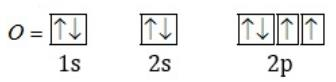Q.24. Nickel atom can lose two electrons to form Ni2+ ion. The atomic number of nickel is 28. From which orbital will nickel lose two electrons.
Ans.
Ni (28): ls2 2s2 2p6 Is2 3p6 3d8 4s2
Ni2+ (26): Is2 2s2 2p6 3s2 3p6 3d8
Hence, to form Ni2+ ion, it will lose 2 electrons from 4s orbital.

Q.25. Which of the following orbitals are degenerate?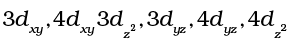Ans.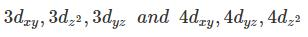Q.26. Calculate the total number of angular nodes and radial nodes present in 3p orbital.
Ans.
For 3p orbital n = 3, l = 1
Number of angular nodes = l = 1
Number of radial nodes = n - l - 1 = 3 - 1 - 1 = 1

Q.27. The arrangement of orbitals on the basis of energy is based upon their (n+l) value. Lower the value of (n+l), lower is the energy. For orbitals having same values of (n+l), the orbital with lower value of n will have lower energy.
I. Based upon the above information, arrange the following orbitals in the increasing order of energy.
(a) 1s, 2s, 3s, 2p
(b) 4s, 3s, 3p, 4d
(c) 5p, 4d, 5d, 4f, 6s
(d) 5f, 6d, 7s, 7p
II. Based upon the above information, solve the questions given below:
(a) Which of the following orbitals has the lowest energy?
4d, 4f, 5s, 5p
(b) Which of the following orbitals has the highest energy?
5p, 5d, 5f, 6s, 6p
Ans.

I.
(a) 1s<2s<2p<3s
(b) 3s<3p<4s<4d
(c) 4d<5p<6s<4f<5d
(d) 7s<5f<6d<7p
II.
(a) 5s (b) 5f

Q.28. Which of the following will not show deflection from the path on passing through an electric field?
Proton, cathode rays, electron, neutron.
Ans.
Neutron, being neutral will not show deflection from the path on passing through an electric field.

Q.29. An atom having atomic mass number 13 has 7 neutrons. What is the atomic number of the atom?
Ans.
Atomic mass number = A = 13. n = 7
As A = n + p p = A — n= 13 —7 = 6
Hence Z = p = 6

Q.30. Wavelengths of different radiations are given below :
λ (A) = 300 nm
λ (B) = 300 µm
λ (C) = 3 nm
λ (D) = 30 Å

Arrange these radiations in the increasing order of their energies.
Ans.
E = hv or = hc/λ or E ∝ 1/λ
λ(A) = 300 nm = 300 x 10-9 m or = 3 x 10-7 m;
λ(B) = 300 x 10-6 m = 3 x 10-4 m
λ(C) = 3 x 10-9 m, λ(D) = 30 x 10-10 m = 30 x 10-9 m
∴ Increasing order of energies is:
B < A < C = D

Q.31. The electronic configuration of valence shell of Cu is 3d104s1 and not 3d94s2.
How is this configuration explained?
Ans.
The valance electronic configuration of Cu is 3d104s1 and not 3d94s1 because in some elements, when the two sub-shells differ slightly in their energies, an electron shifts from a sub-shell of lower energy (4s) to a sub-shell of a higher energy (3d). It has been found that there is extra stability associated with these electronic configuration.

Q.32. The Balmer series in the hydrogen spectrum corresponds to the transition from n1 = 2 to n2 = 3, 4,......... . This series lies in the visible region. Calculate the wave number of line associated with the transition in Balmer series when the electron moves to n = 4 orbit. (RH = 109677 cm–1)
Ans.

Here n= 2, n= 4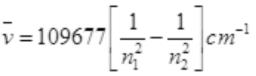for n= 2, n= 4 transition in Balmer series.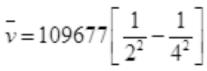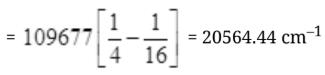Q.33. According to de Broglie, matter should exhibit dual behaviour, that is both particle and wave like properties. However, a cricket ball of mass 100 g does not move like a wave when it is thrown by a bowler at a speed of 100 km/h. Calculate the wavelength of the ball and explain why it does not show wave nature.
Ans.
According to be Broglie
The wavelength λ =h/mv
m = 100 g = 0.1 kg.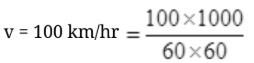h = 6.626×10–34 Js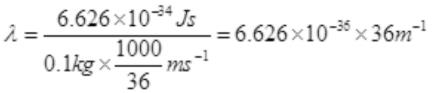λ = 238.5 x 10-36 m-1
Since the wavelength is too small to be detected, so, it does not show wave nature.

Q.34. What is the experimental evidence in support of the idea that electronic energies in an atom are quantized?
Ans.
Classical mechanics fails when applied to microscopic objects like electrons, atoms and molecules, etc.
This is because the classical mechanics ignores the concept of dual nature of matter.
The branch of science that takes into account this dual behaviour of matter is called quantum mechanics.

Q.35. Out of electron and proton which one will have, a higher velocity to produce matter waves of the same wavelength? Explain it.
Ans.
λ = h/mv = h/p
Where m is the mass of the particle and v is the velocity of the particle. Lighter the mass higher will be the velocity of the particle.
Electron will have higher velocity.

Q.36. A hypothetical electromagnetic wave is shown in Figure. Find out the wavelength of the radiation.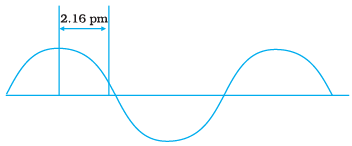Ans.
Wavelength: It may be defined as the distance between two neighboring crests or troughs of a wave as shown. It is denoted by λ.
λ = 4 x 2.16 pm = 8.64pm

Q.37. Chlorophyll present in green leaves of plants absorbs light at 4.620 × 1014 Hz.
Calculate the wavelength of radiation in nanometer. Which part of the electromagnetic spectrum does it belong to?
Ans.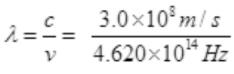= 0.6494 x 10-6 m = 649.4 nm
It belongs to the visible light of the spectrum.

Q.38. What is the difference between the terms orbit and orbital?
Ans.

 Orbit Orbital 1. An orbit is a well-defined circular path around the nucleus in which the electrons revolve. 1. An orbital is the three-dimensional space around the nucleus within which the probability of finding an electron is maximum (upto 90%) 2. It represents the planar motionof an electron around the nucleus. 2. It represents the three dimensional motion of an electron around the nucleus. 3. All orbits are circular and disclike. 3. Different orbitals have different shapes, i.e. s-orbitals are spherically symmetrical, p-orbitals are dumb-bell shaped and so on.

Q.39. Table-tennis ball has a mass 10 g and a speed of 90 m/s. If speed can be measured within an accuracy of 4% what will be the uncertainty in speed and position?
Ans.
Uncertainty in the speed of ball = (90 x 4)/100 = 360/100 = 3.6 ms-1
Uncertainty in position = h/hπmΔv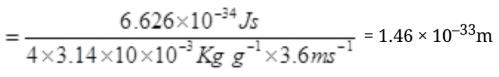Q.40. The effect of uncertainty principle is significant only for motion of microscopic particles within an accuracy of 4% what will be the uncertainty in speed and position?
Ans. Δv.Δx = h/4πm
Δv.Δx = (h/(4 x 3.14 x m))
If we consider mas as 10-6 kg (1 milligram)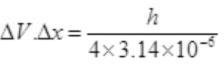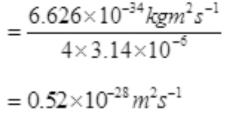Q.41. Hydrogen atom has only one electron, so mutual repulsion between electrons is absent. However, in multielectron atoms mutual repulsion between the electrons is significant. How does this affect the energy of an electron in the orbitals of the same principal quantum number in multielectron atoms?
Ans.
The energy of an electron in a hydrogen atom is determined solely by the principal quantum number. Thus, the energy of the orbitals increases as follows:
1s < 2s = 2p < 3s = 3p = 3d< 4s = 4p = 4d = 4f < (2.23).
The energy of an electron in a multielectron atom, unlike that of the hydrogen atom, depends not only on its principal quantum number (shell), but also on its azimuthal quantum number (subshell). That is, for a given principal quantum number, s, p, d, f ... all have different energies.

MATCHING TYPE

In some of the following questions, one option of left column may be correlated to more than one option in the right column.
Q.42. Match the following species with their corresponding ground state electronic configuration.

 Atom / Ion Electronic configuration (i) Cu (a) 1s2 2s2 2p6 3s2 3p6 3d10 (ii) Cu2+ (b) 1s2 2s2 2p6 3s2 3p6 3d10 4s2 (iii) Zn2+ (c) 1s2 2s2 2p6 3s2 3p6 3d10 4s1 (iv) Cr3+ (d) 1s2 2s2 2p6 3s2 3p6 3d9 (e) 1s2 2s2 2p6 3s2 3p6 3d3

Ans. (i) →(c); (ii) →(d); (iii) → (a); (iv) → (e)

Q.43. Match the quantum numbers with the information provided by these.

 Quantum number Information provided (i) Principal quantum number (a) orientation of the orbital (ii) Azimuthal quantum number (b) energy and size of orbital (iii) Magnetic quantum number (c) spin of electron (iv) Spin quantum number (d) shape of the orbital

Ans. (i) →(b); (ii) →(d); (iii) →(a); (iv) →(c)

Q.44. Match the following rules with their statements:

 Rules Statements (i) Hund’s Rule (a) No two electrons in an atom can have the same set of four quantum numbers. (ii) Aufbau Principle (b) Half-filled and completely filled orbitals have extra stablity. (iii) Pauli Exclusion Principle (c) Pairing of electrons in the orbitals belonging to the same subshell does not take place until each orbital is singly occupied. (iv) Heisenberg’s Uncertainty Principle (d) It is impossible to determine the exact position and exact momentum of a subatomic particle simultaneously. (e) In the ground state of atoms, orbitals are filled in the order of their increasing energies.

Ans. (i) →(c); (ii) →(e); (iii) →(a); (iv) →(d)

Q.45. Match the items given in Column I with the items given in Column II:

 Column I Column II (i) X-rays (a) ν = 100 – 104 Hz (ii) UV (b) ν = 1010 Hz (iii) Long radio waves (c) ν = 1016 Hz (iv) Microwave (d) ν = 1018 Hz

Ans. (i)→(d); (ii) →(c); (iii) → (a); (iv) → (b)

Q.46. Match the items given in Column I with the items given in Column II:

 Column I Column II (i) Photon (a) Value is 4 for N shell (ii) Electron (b) Probability density (iii) ψ2 (c) Always positive value (iv) Principal quantum number n (d) Exhibits both momentum and wavelength

Ans. (i) →(d); (ii) →(d); (iii) →(b), (c); (iv) →(a), (c)

Q.47. Match species given in Column I with the electronic configuration given in Column II.

 Column I Column II (i) Cr (a) [Ar]3d84s0 (ii) Fe2+ (b) [Ar]3d104s1 (iii) Ni2+ (c) [Ar]3d64s0 (iv) Cu (d) [Ar] 3d54s1 (e) [Ar]3d64s2

Ans. (i) →(d); (ii) →(c); (iii) →(a); (iv) →(b)

ASSERTION AND REASON TYPE QUESTIONS

In the following questions a statement of Assertion (A) followed by a statement of Reason (R) is given. Choose the correct option out of the choices given below each question.
Q.48. Assertion (A): All isotopes of a given element show the same type of chemical behaviour.
Reason (R): The chemical properties of an atom are controlled by the number of electrons in the atom.
(1) Both A and R are true and R is the correct explanation of A.
(2) Both A and R are true but R is not the correct explanation of A.
(3) A is true but R is false.
(4) Both A and R are false.

Ans. (1)
All isotopes have same atomic number means same number of electrons and they bear similar chemical properties.

Q.49. Assertion (A): Black body is an ideal body that emits and absorbs radiations of all frequencies.
Reason (R): The frequency of radiation emitted by a body goes from a lower frequency to higher frequency with an increase in temperature.
(i) Both A and R are true and R is the correct explanation of A.
(ii) Both A and R are true but R is not the explanation of A.
(iii) A is true and R is false.
(iv) Both A and R are false.
Ans.  (2)
Solution.
The ideal body, which emits and absorbs radiations of all frequencies, is called a black body and the radiation emitted by such a body is called black body radiation. The exact frequency distribution of the emitted radiation (i.e. intensity versus frequency curve of the radiation) from a black body depends only on its temperature. At a given temperature, intensity of radiation emitted increases with decrease of wavelength, reaches a maximum value at a given wavelength and then starts decreasing with further decrease of wavelength.

Q.50. Assertion (A): It is impossible to determine the exact position  and exact momentum of an electron simultaneously.
Reason (R): The path of an electron in an atom is clearly defined.
(1) Both A and R are true and R is the correct explanation of A
(2) Both A and R are true and R is not the correct explanation of A
(3) A is true and R is false
(4) Both A and R are false

Ans. (3)
Solution. The effect of Heisenberg Uncertainty Principal is significant only for motion of microscopic objects and is negligible for that of macroscopic objects.

Q.51. What is photoelectric effect? State the result of photoelectric effect experiment that could not be explained on the basis of laws of classical physics. Explain this effect on the basis of quantum theory of electromagnetic radiations.
Ans.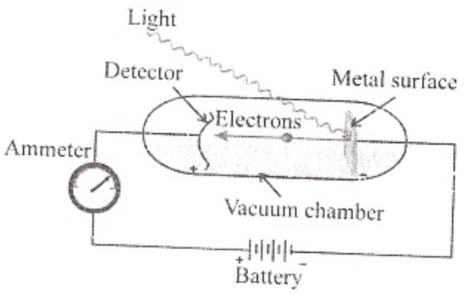Photoelectric effect: When certain metals are exposed to a beam of light, the electrons are ejected from the metal. This phenomenon is called photoelectric effect. The electrons ejected are called photoelectrons.
The results of this experiment were:
1. The electrons are ejected from the metal surface only as the beam of light strikes the surface.
2. The number of electrons ∝ intensity of radiation.
3. For each metal; there is a minimum frequency, v0 below which photoelectric effect is not observed.
4. K.E. of electrons ∝ frequency of light.
Explanation of photoelectric effect on the basis of quantum theory: When a photon of sufficient energy strikes an electron in the atom of the metal, it transfers its energy to electron and the electron is ejected without delay. Greater the energy of the photon, greater will be the kinetic energy of the ejected electron.

Q.52. Threshold frequency, ν0 is the minimum frequency which a photon must possess to eject an electron from a metal. It is different for different metals. When a photon of frequency 1.0×1015 s–1 was allowed to hit a metal surface, an electron having 1.988 × 10–19 J of kinetic energy was emitted. Calculate the threshold frequency of this metal. Show that an electron will not be emitted if a photon with a wavelength equal to 600 nm hits the metal surface.
Ans.

hv = h0 + K.E.
hv0 = hv - K.E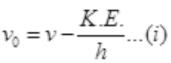v0 = 1.0 x 1015 s-1
K.E = 1.988 x 10-19 J, h = 6.626 x 10-34
From (i) we have,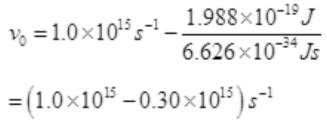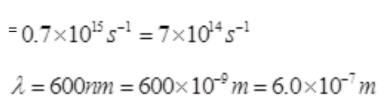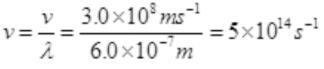Thus v<v0, hence, no electron will be emitted.

Q.53. When an electric discharge is passed through hydrogen gas, the hydrogen molecules dissociate to produce excited hydrogen atoms. These excited atoms emit electromagnetic radiation of discrete frequencies which can be given by the general formula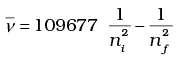What points of Bohr’s model of an atom can be used to arrive at this formula Based on these points derive the above formula giving description of each step and each term.
Ans.
The following points of Bohr’s model of an atom can be used to arrive at the given formula:
(i) Electrons revolve around the nucleus in circular orbits with fixed values of energy.
(ii) When electron jumps from one orbit to another, energy is emitted or absorbed.
Derivation of the given formula: The energy of electron in the nth stationary state is given by
Where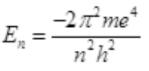m = mass of electron
e = charge on electron
h = Planck's constant
When electron jumps from outer orbit n2 to inner orbit n1, the difference of energy (ΔE) is emitted.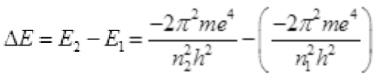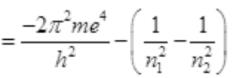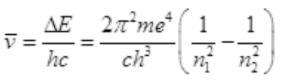By putting values of π, m, c, h and e we get,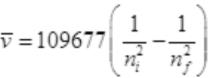Q.54. Calculate the energy and frequency of the radiation emitted when an electron jumps from n = 3 to n = 2 in a hydrogen atom.
Ans.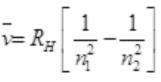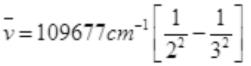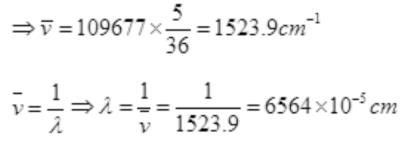λ = 6564 x 10-7m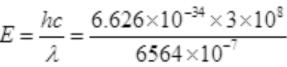=3.028 x 10-19 J

Q.55. Why was a change in the Bohr Model of atom required? Due to which important development (s), concept of movement of an electron in an orbit was replaced by, the concept of probability of finding electron in an orbital? What is the name given to the changed model of atom?
Ans.  In view of the shortcoming of the Bohr’s model, attempts were made to develop a more suitable and general model for atoms. Two important developments which contributed significantly in the formulation of such a model were:
(i) Dual behavior of matter,
(ii) Heisenberg uncertainty principle.
Werner Heisenberg, a German physicist in 1927, stated uncertainty principle which is the consequence of duel behaviour of matter and radiation. One of the important implication of the Heisenberg Uncertainty Principle is that it rules out existence of definite paths or trajectories of electrons and other similar particles.
Quantum mechanics is the theoretical science that deal with the study of the motions of the microscopic objects that have both observable wave like and particle like properties. The name of the changed model of atom is Quantum Mechanical Model of atom.

Offer running on EduRev: Apply code STAYHOME200 to get INR 200 off on our premium plan EduRev Infinity!

170 docs

,

,

,

,

,

,

,

,

,

,

,

,

,

,

,

,

,

,

,

,

,

;